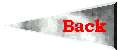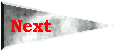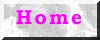I. Phonology

I.1. Alphabet

a, à, b, c, ç, d, e, è, f, g, h, i, ì, j, l, m, n, q, r, s, sh, t, u, v, w, x, y

I.2. Vocalism:

The monophtongs relating to the monophtongs are:

a [a], à [^], e [e], i [i], ì [I], u [U], y [y]

Remarks:

-) The monophtongs signified by (`) only occur in certain positions and are marked here solely to be recognizable. The rules are: <à> is used in front of the unvoiced plosives (c, q, t) and geminate consonants (rr, ll, etc.). As regards the syllable structure of these words the vowels stand in closed syllables; here the consonants after the sound are slightly longer than in normal position; e.g. cràte = /kr^t;'1/ (to welcome, to greet), but: crene /kre'n1/ (to swell); gàtet = /g^t;1t/ (ban); càllna = /k^L;'n7/ (mountain) (with [L] being an allophone of [l] conditioned by [^]). Furthermore <ì> occurs before c and x; e.g. crìxe /krI'X1/ (to grow). For <è> see point b).
-)  occurs in final position after consonants and vowels; e.g. like above in cna'e /kn7'1/ (to give birth to).

b) Diphtongs:
Clalian knows only two proper diphtongs: <au> and <ai> ([
7U] resp. [7I]). <aw>, <ew> etc. occuring as digraphs have a strongly rounded [U] approximating a bilabial fricative [B] without actually forming an occlusion. The digraphs <ie> resp. <iè> indicate a combination of the half-vowel /j/ and an [e] resp. [E]. Here <iè> only occurs in front of <x> (= [X]).

c) Half-vowels:
The half-vowels /j/ and /w/ are marked by normal letters. In final position after consonants <j> and <w> become [
I] resp. [f] when followed by another conconants (as happens in the verb); e.g. darwe (sleep) Þ darf'te (he's sleeping); fije (to write) Þ fij'na [fi:n7] (I write).

I.1. Consonants:
Apart from a few exceptions the graphemes mark simply the corresponding sounds b, d, m, etc. Special graphemes and their sounds: <c> = [
k], <ç> = [tS]; <q> = [q] (velar /k/; not /kw/!); <sh> = [S]. <x> always marks /X/, never /ç/.